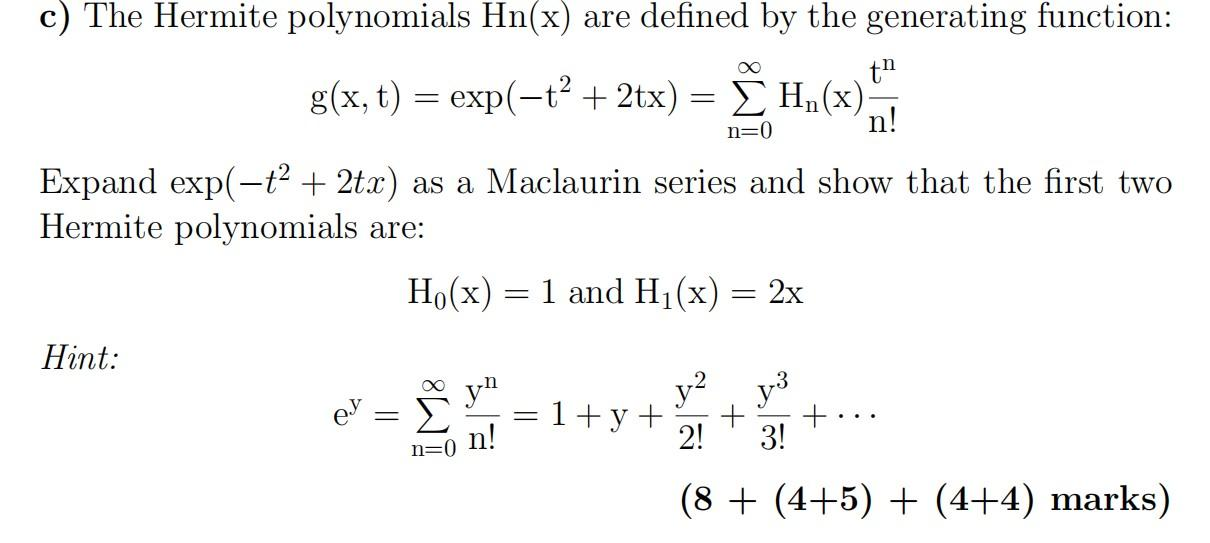# Question c) The Hermite polynomials Hn(x) are defined by the generating function: th = ΣΗ() n! 2 g(x, t) = exp(-+? + 2tx) = Ï H.(x) = n=0 Expand exp(-ť? + 2tx) as a Maclaurin series and show that the first two Hermite polynomials are: H.(x) = 1 and H1(x) 2x = Hint: yn y2 you ey IM8 = 1+ y + + 2! n=on! 3! (8 + (4+5) + (4+4) marks)